Heat Capacity of a system is defined as the amount of heat required to raise the temperature of a system by 1oC.where, coefficient C is called the heat capacity.

C is directly proportional to the amount of substance.It is the heat capacity for 1 mole of the substance.

### Molar heat capacity

It is defined as the quantity of heat required to raise the temperature of a substance by 1o (kelvin or Celsius).

### Specific Heat Capacity

It is defined as the heat required to raise the temperature of one unit mass of a substance by 1o (kelvin or Celsius).

q = C × m × ∆T

where m = mass of the substance

∆T = rise in temperature.

### Relation between Cp and Cv for an Ideal Gas

At constant volume heat capacity = Cv

At constant pressure heat capacity = Cp

At constant volume qv = Cv ∆T = ∆U

At constant pressure qv = Cp ∆T = ∆H

For one mole of an ideal gasOn substituting the values of ∆H and ∆U, the equation is modified as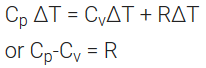## Measurement of ∆U and ∆H: Calorimetry

Determination of ∆U: ∆U is measured in a special type of calorimeter, called bomb calorimeter.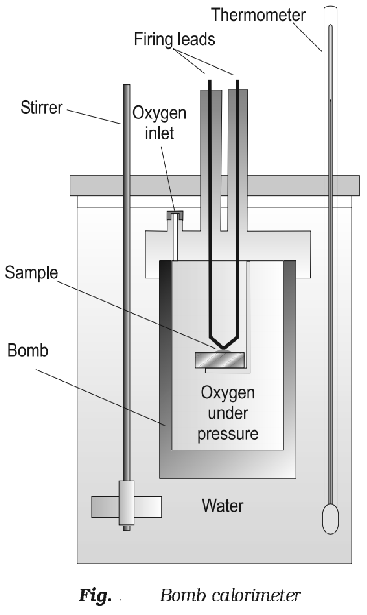### Working with calorimeter:

The calorimeter consists of a strong vessel called (bomb) which can with stand very high pressure. It is surrounded by a water bath to ensure that no heat is lost to the surrounding.

### Procedure:

A known mass of the combustible substance is burnt in the pressure of pure dioxygen in the steel bomb. Heat evolved during the reaction is transferred to the water and its temperature is monitored.

During the process volume remains constant

∆V = 0

Temperature change of the calorimeter is converted to qv.

The value of ∆U can be calculated using the formula.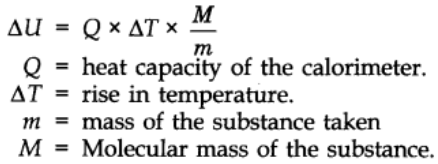### Determination of ∆H:

At constant pressure heat evolved or absorbed is ∆qp is equal to the heat of reaction or enthalpy of reaction ∆rH.

rH = (Sum of enthalpies of products) – (Sum of enthalpies of reactants)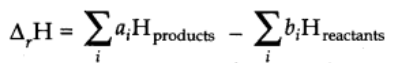ai and bi are the stoichiometric coefficients.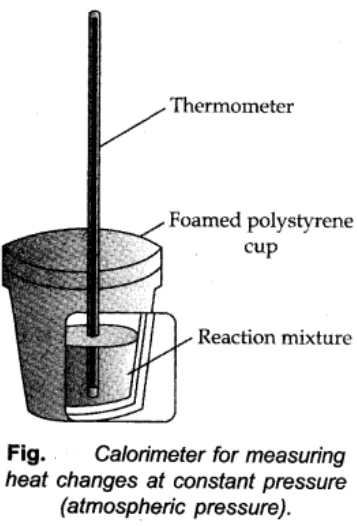Standard enthalpy of reactants: When reaction takes place at the standards state of substance the enthalpy of reaction is known as standard enthalpy. It is denoted by ∆H.